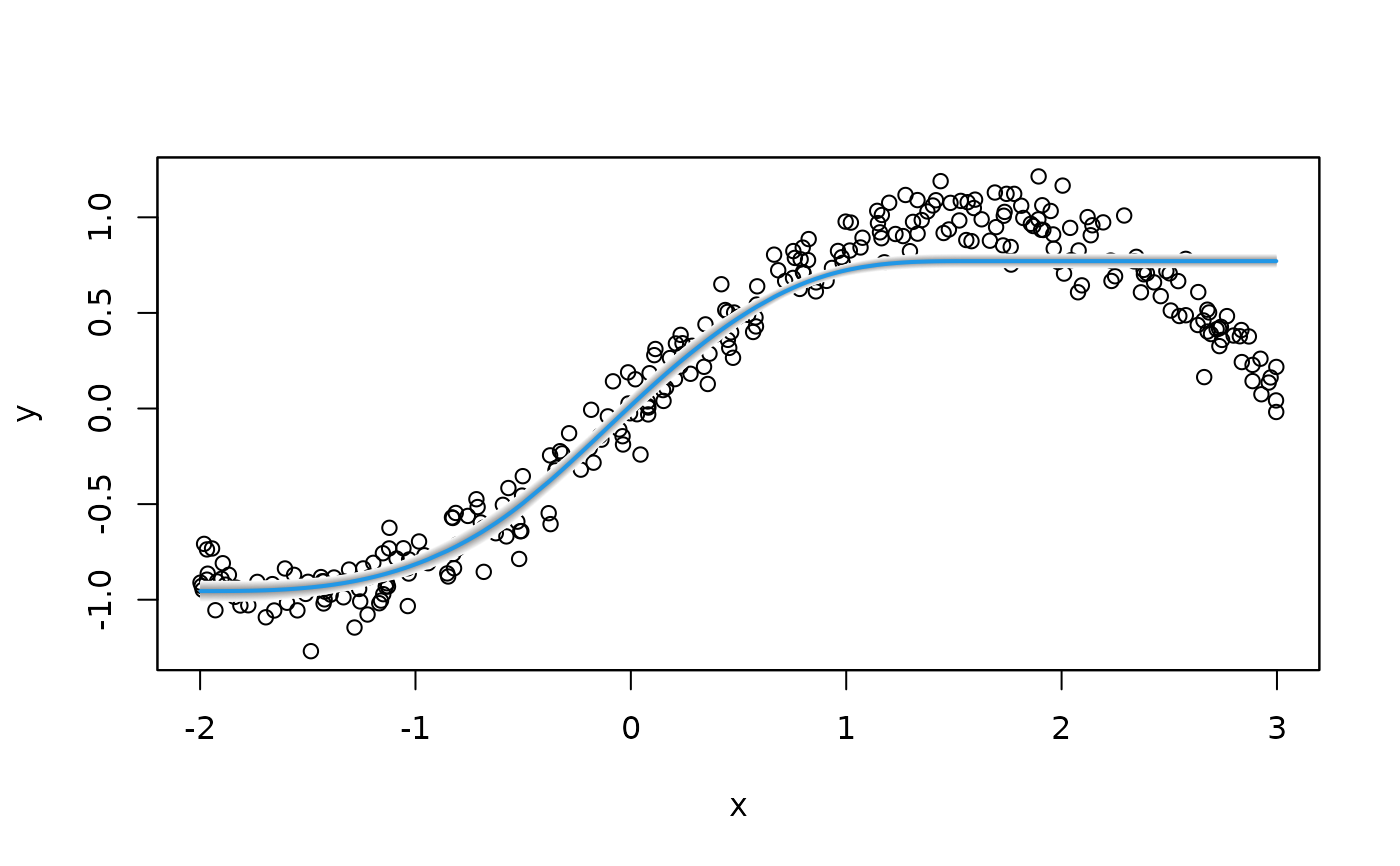The function sets up a smooth term for shape constraint estimation of P-spline model terms. Note that this currently only works using boosting and backfitting.

# S3 method for ms.smooth.spec
smooth.construct(object, data, knots, ...)

## Arguments

object

Either a smooth specification object, or object of class "bamlss", "bamlss.frame", "bamlss.formula" or "bamlss.terms". For smooth specification objects, see function smooth.construct.

data

A data frame or list, see also see function smooth.construct.

knots

See function smooth.construct.

...

Arguments passed to the smooth term constructor functions.

## Value

See function see smooth.construct.

bamlss.frame, bamlss.formula, bamlss, smooth.construct.

## Examples

if (FALSE) ## Generate some data.
set.seed(123)

n <- 300
x <- runif(n, -2, 3)
y <- sin(x) + rnorm(n, sd = 0.1)

## Increasing: constr = 1.
## Decreasing: constr = 2.
b <- bamlss(y ~ s2(x,bs="ms",xt=list(constr=1)),
data = d, sampler = FALSE, optimizer = opt_bfit)
#> Error in bamlss.model.frame(formula, data, family, weights, subset, offset,     na.action, specials, contrasts): object 'd' not found

## Predict and plot.
p <- predict(b, model = "mu")